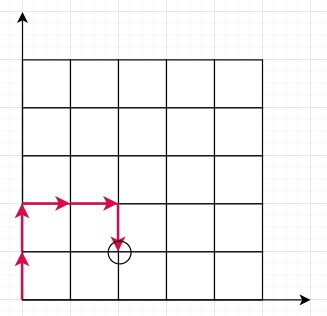# Program to check robot can reach target position or not in Python

Suppose we have a robot, that is currently sitting in at position (0, 0) (Cartesian plane). If we have list of its moves that it can make, containing N(North), S(South), W(West), and E(East). We have to check whether it can reach at destination coordinate (x, y).

So, if the input is like moves = ['N','N','E','E','S'], (x,y) = (2,1), then the output will be True,To solve this, we will follow these steps −

• temp_coord := [0,0]
• for each move in moves, do
• if move is same as "N", then
• temp_coord := temp_coord + 1
• otherwise when move is same as "S", then
• temp_coord := temp_coord - 1
• otherwise when move is same as "E", then
• temp_coord := temp_coord + 1
• otherwise when move is same as "W", then
• temp_coord := temp_coord - 1
• return True when temp_coord is same as coord and temp_coord is same as coord otherwise false.

Let us see the following implementation to get better understanding −

## Example

Live Demo

class Solution:
def solve(self, moves, coord):
temp_coord = [0,0]
for move in moves:
if move == "N":
temp_coord += 1
elif move == "S":
temp_coord -= 1
elif move == "E":
temp_coord += 1
elif move == "W":
temp_coord -= 1
return temp_coord == coord and temp_coord == coord
ob = Solution()
moves = ['N','N','E','E','S']
coord = [2,1]
print(ob.solve(moves, coord))

## Input

['N','N','E','E','S'], [2,1]

## Output

True